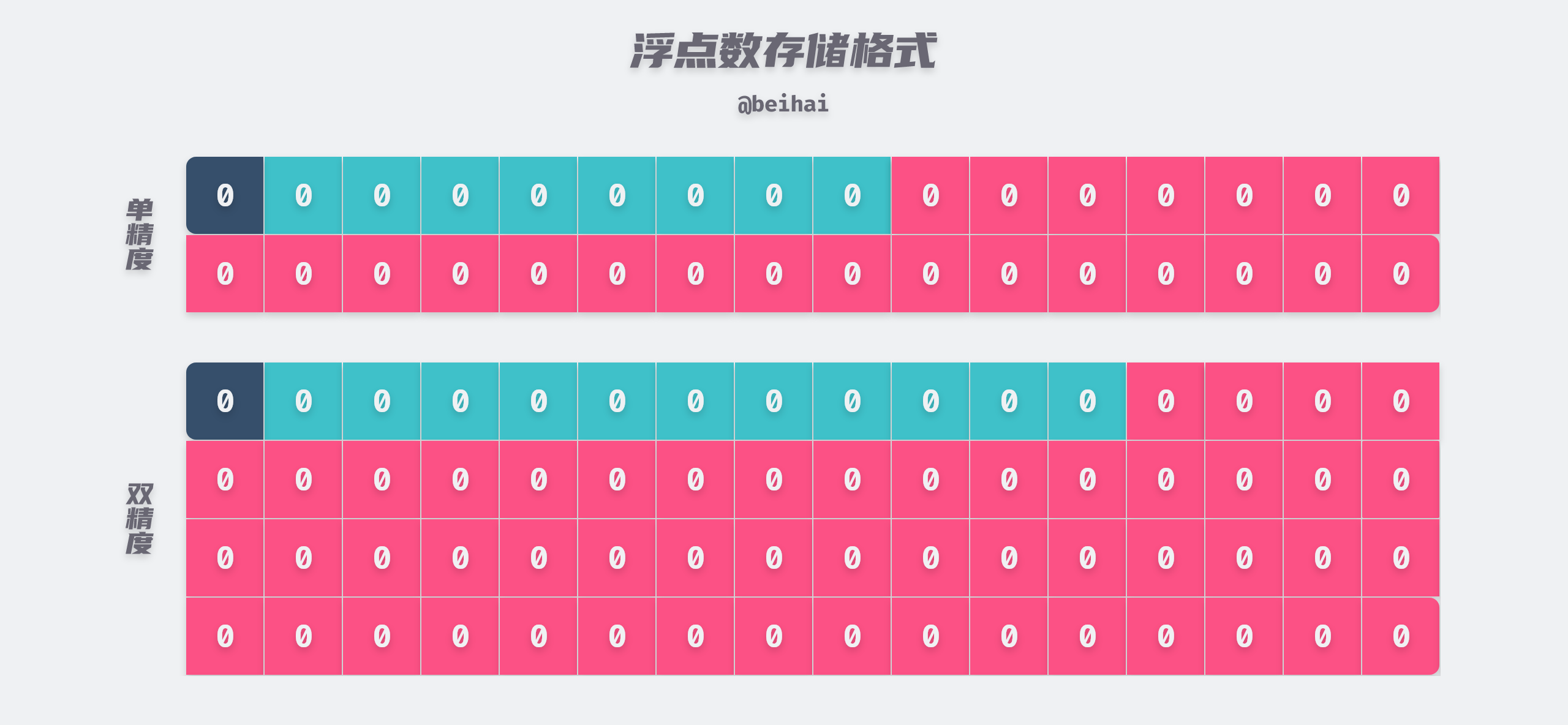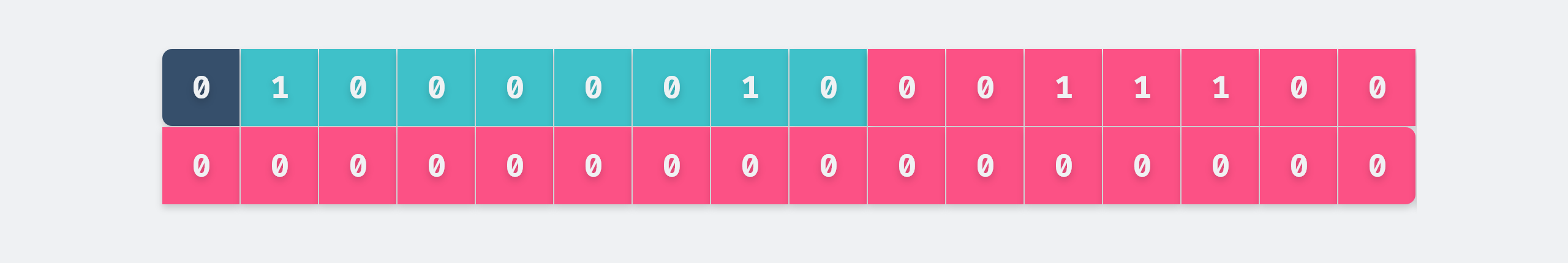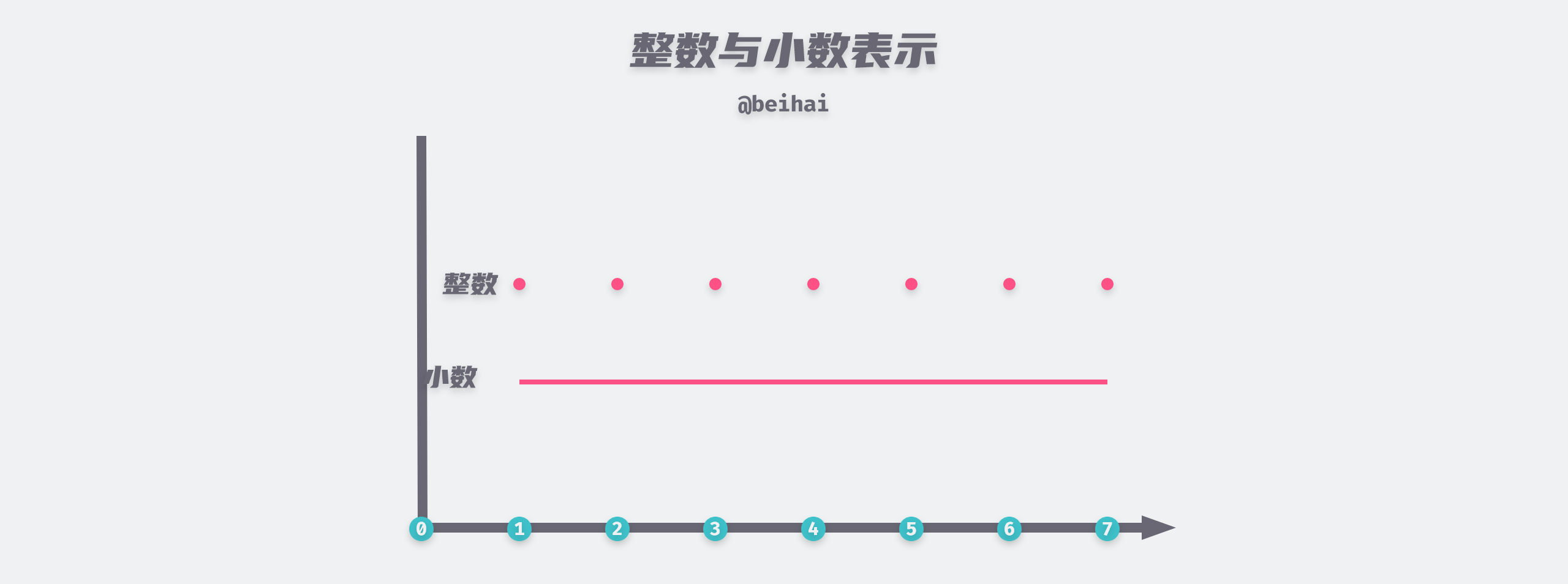The IEEE standard for binary floating-point arithmetic (IEEE 754) is the most widely used standard for floating-point arithmetic since the 1980s and is used by many CPUs and floating-point operators. However, this floating-point representation also poses certain accuracy problems, which we will discuss.

IEEE 754 provides four precision specifications, of which single-precision floating-point and double-precision floating-point are the most commonly used types, and most programming languages today such as C, Go, Java, JavaScript, etc. provide the standard floating-point format. The IEEE single-precision format has 24 valid binary bits and occupies a total of 32 bits, while the IEEE double-precision format has 53 valid binary bits and occupies a total of 64 bits.

## Storage Format

A floating-point number divides consecutive binary bits into three parts of a specific width: the sign field, the exponent field, and the mantissa field, where the saved values are used to represent the positive and negative sign, exponent, and mantissa of a given binary floating-point number, respectively, and to express the given value through the mantissa and the adjustable exponent. In a single-precision floating-point number, the sign bit occupies the highest bit and is used to indicate whether the floating-point number is positive or negative, with 0 being positive and 1 being negative; the exponent bit occupies the next 8 bits; and finally, the trailing 23 bits. A double precision floating point number is divided into 1 bit for the sign, 11 bits for the exponent, and 52 bits for the mantissa. There is no difference between these two representation formats except that the bit lengths are not the same.Let’s take a single-precision floating-point number as an example. The exponent bit takes up 8 bits of space to represent the exponent size, but its actual value is [-126,127], and -127 and 128 are used as special values. The exponent bit also has an offset, and the value stored in the exponent bit is the actual value of the exponent plus a fixed offset. The advantage of this is that all exponent values can be represented as unsigned integers of length N, making it easier to compare the exponent size of two floating-point numbers. The offset is 127 for single-precision floating-point numbers and 1023 for double-precision floating-point numbers, so if the actual value of the exponent is 10, then the value stored in the exponent bit is 127 + 10 = 127.

For details on the storage format of floating point numbers, see wiki IEEE 754

Suppose we want to store a single-precision floating-point number 9.75, then we need to convert the decimal number to binary 1001.11, and then convert the binary number to an exponent with a base of 2. 1001.11 = 1.00111 × 23, where 1.00111 is the mantissa and 3 is the exponent. We find that the highest digit of the mantissa is always 1, so we can store it by omitting the 1 before the decimal point, thus freeing up a binary digit to hold more of the mantissa. Thus we actually have a 24-bit mantissa with a 23-bit mantissa field. In this example the trailing digit is 00111 and the trailing digit is complemented by 0. The exponent digit is stored as 3 + 127 = 130, which is 10000010. The final 9.75 single-precision floating-point number is stored as follows.## Accuracy issues

### Maximum Significant Digits

The valid numbers for single and double precision floating point numbers are the stored 23 and 52 binary bits, respectively, plus the leftmost unstored 1., which can ultimately represent 24 and 53 bits. So the corresponding formula for calculating the final significant digit is as follows.

$$lg2^{24} ≈ 7.22$$

$$lg2^{53} ≈ 15.95$$

From the above calculations, single- and double-precision floating-point numbers are guaranteed to have 7 and 15 decimal significant digits. Note that the valid number includes the integer part, and the maximum valid number is only the longest decimal decimal number that can be represented by a floating-point number; the exact representation depends on whether the number can be represented in binary form.

### Binary conversion

Before storing floating point numbers we need to convert decimal numbers to binary numbers, but most decimal numbers cannot be represented exactly in binary. Suppose we want to represent the decimal number 0.1 as binary, then the calculation process is as follows.

  1 2 3 4 5 6 7 8 9 10  0.1 x 2 = 0.2 取整数位 0 得 0.0 0.2 x 2 = 0.4 取整数位 0 得 0.00 0.4 x 2 = 0.8 取整数位 0 得 0.000 0.8 x 2 = 1.6 取整数位 1 得 0.0001 0.6 x 2 = 0.2 取整数位 1 得 0.00011 0.2 x 2 = 0.4 取整数位 0 得 0.000110 0.4 x 2 = 0.8 取整数位 0 得 0.0001100 0.8 x 2 = 1.6 取整数位 1 得 0.00011001 0.6 x 2 = 1.2 取整数位 1 得 0.000110011 ... 

What we end up with is an infinite loop of binary decimals 0.000110011 …, and even if we take the decimal part to infinity, the value can only be infinitely close to 0.1, but not to 0.1. Since binary numbers can only represent polynomial sums to the power of 2 precisely, many decimals lose precision in the process of decimal conversion. The precision is already lost in this process.

### Value range and rounding error

The section on floating point storage formats shows that a floating point number is calculated as follows

• ± Tail value × 2 Exponential value

Since the value range of the tail value is [1,2) (including the omitted 1.) and the value range of the exponent value is [-126,127], the value range of a single-precision floating-point number is theoretically ±[1,2) × 2[-126,127]. However, in practice both the trailing and exponential values are stored in binary form, and they can only represent a finite number of states. The difference between decimals and integers is that integers between [1,7] have only 7 finite digits and we only need to store 7 different states in the computer; however, decimals between [1,7] are a continuous line, and no matter how long the valid bits of a floating point number are, we can never represent an infinite number of decimals with finite states.The floating point representation also divides the decimals into a dense distribution of dots, so during numerical calculations, the resulting data is approximated using the relevant rounding rules, which can lead to errors in the calculation results. the default rounding method for the IEEE floating point format is rounding to even , which tries to find a closest match for rounding, and in the case of intermediate value, then it will round to the even value. Thus, it will round 1.4 to 1 and 1.6 to 2, but both 1.5 and 2.5 will be rounded to 2.

When rounding, the last digit from 1 to 9 is rounded to 1, 2, 3, and 4, while 9, 8, 7, and 6 are rounded, and 5 is left alone. If we round to the nearest 5 each time, we will accumulate large deviations when doing some statistics with large amounts of data. If we use the strategy of rounding to even numbers, in most cases, the probability of rounding off and rounding in 5 is about the same, and the bias in the statistics will be smaller accordingly.

Rounding errors are also present in the process of binary conversion, if we want to store the decimal decimal 0.1, then the end of the floating point number is represented as 100110011001100110011001101 and the last binary bit 1 is rounded to get it.

### Irregular distribution

In the storage method of floating-point numbers, if the exponent bits are constant, then the interval between two adjacent trailing bits is certain, but the interval between two adjacent exponent fields is different. Let’s assume that the trailing part is all 0 and the exponent part is 10000000, which means the single precision floating point number is 2.0; add 1 to the exponent part and change it to 10000001, which means the single precision floating point number is 4.0; add 1 to the exponent part and change it to 10000010, which means the single precision floating point number is 8.0. The interval between the floating point numbers in the range [2.0, 4.0) is 2/223, while the interval between the floating point numbers in the range [4.0, 8.0) is 4/223.

The interval between floating point numbers is doubled for every 1 added to the exponential part.The larger the exponent, the more sparse the interval between floating-point numbers becomes. When the exponent value after offset reaches 150, the interval between two adjacent single-precision floating-point numbers is already 1 and it is no longer possible to save the number after the decimal point.

## Summary

The IEEE 754 standard also specifies a decimal representation without loss of precision, a data type called Decimal, which uses strings to store the fractional part of the data, but this data type also wastes some of the storage space. The issue of precision in floating-point numbers is based on a compromise between performance and accuracy, and when this standard was first defined, memory was a scarce computational resource, so there was a preference for this representation at the expense of precision.